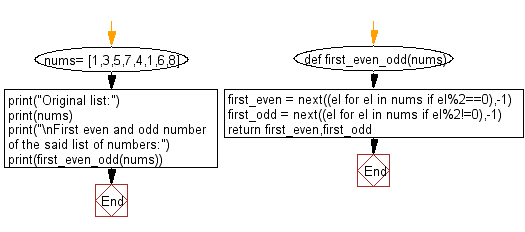﻿ Python: First even and odd number in a given list of numbers - w3resource# Python: First even and odd number in a given list of numbers

## Python List: Exercise - 137 with Solution

Write a Python program to find a first even and odd number in a given list of numbers.

Sample Solution:

Python Code:

``````def first_even_odd(nums):
first_even = next((el for el in nums if el%2==0),-1)
first_odd = next((el for el in nums if el%2!=0),-1)
return first_even,first_odd

nums= [1,3,5,7,4,1,6,8]
print("Original list:")
print(nums)
print("\nFirst even and odd number of the said list of numbers:")
print(first_even_odd(nums))
```
```

Sample Output:

```Original list:
[1, 3, 5, 7, 4, 1, 6, 8]

First even and odd number of the said list of numbers:
(4, 1)
```

Flowchart:## Visualize Python code execution:

The following tool visualize what the computer is doing step-by-step as it executes the said program:

Python Code Editor:

Have another way to solve this solution? Contribute your code (and comments) through Disqus.

What is the difficulty level of this exercise?

Test your Python skills with w3resource's quiz

﻿

## Python: Tips of the Day

```print(2_000_000)
```2000000